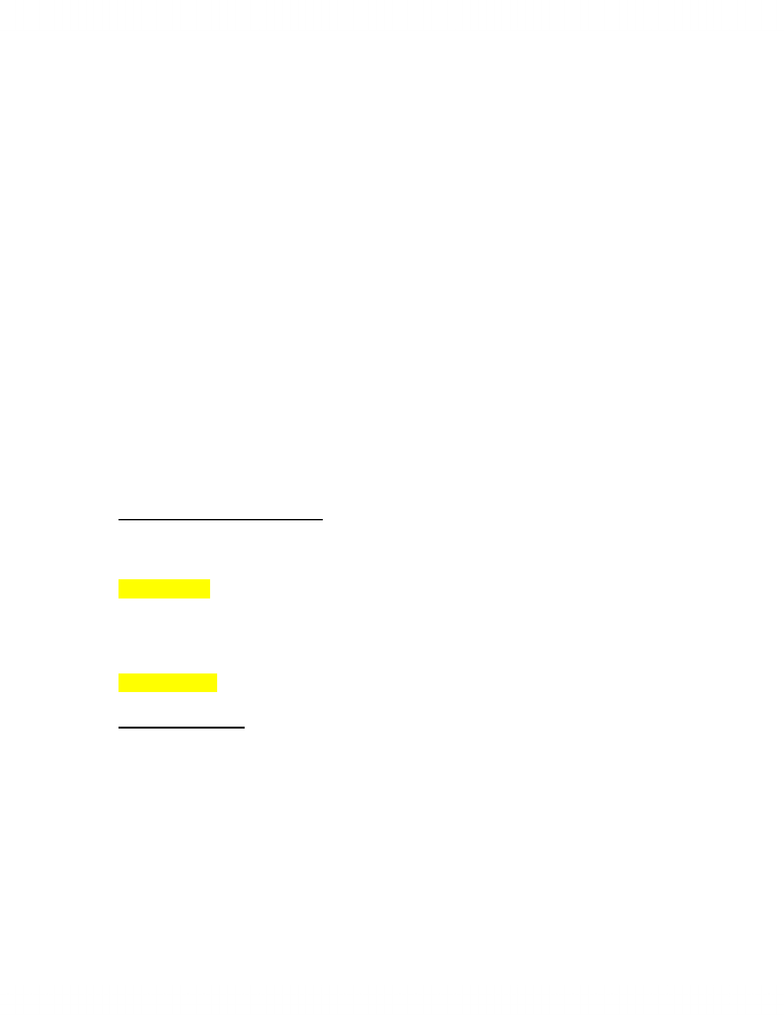# CHEM 1310 Lecture Notes - Jmol, Dihydrogen Cation, Joule

59 views3 pages
School
Department
Course
ProfessorQ. How much heat is required to bring H2O in the form of ice at 0 C to steam at 100 C?
A.
Step 1: melt ice fusion
o Turns to liquid
Step 2: heat
o Turns to H2O at 100 C
Phase change from liquid to gas vaporization
Q = quantity of heat
o =∆Hfus + ∆H + ∆Hvap
o =step 1 + step 2 + step 3
∆H = q = mc∆T
Solve:
o Hfus = 6.02 kJ/mol
o Hvap = 40.7 kJ/mol
o Look these up in table of values
o T = mCspecT
o =18.02g/mol(4.184 J)(100 K)
o =7539.6 J/mol = 7.54 kJ/mol
o Q = step 1 + step 2 + step 3
=6.02 kJ/mol + 7.54 kJ/mol + 40.7 kJ/mol = 54.2 kJ/mol needed
Standard Enthalpy of Formation
Hᵒf
A substances enthalpy change in going from the standard state to the reference state
The value is 0 if the substance is a pure element in the reference state or it is the ion H+
Standard state - the solid or liquid of the pure element or compound when the pressure is 1
bar - commonly found in table format - 1 bar, 298 K
Pure + crystalline = solids
Liquids = normal
Gases = assumed ideal
Reference state - the most stable form (state) of the element or compound
Formation Reaction
Makes mol of cpd/product of interest
Make from standard state or elemental reference
States standard (reference) temperature, pressure + concentrated
Maybe endothermic or exothermic
Hᵒf example:
Hᵒf [C, graphite] = 0 = 0 reference, standard
Hᵒf [C, diamond] = 1.895 kJ/mol = standard state but not reference state
Hᵒf [N2] = 0 = 0 reference and standard state
May calculate using Hess's Law (Hᵒf) or look up in tables
Unlock document

This preview shows page 1 of the document.
Unlock all 3 pages and 3 million more documents.

## Document Summary

Step 1: melt ice fusion: turns to liquid. Step 2: heat: turns to h2o at 100 c. Phase change from liquid to gas vaporization. Q = quantity of heat: = hfus + h + hvap, =step 1 + step 2 + step 3. Solve: hfus = 6. 02 kj/mol, hvap = 40. 7 kj/mol, look these up in table of values, t = mcspec t, =18. 02g/mol(4. 184 j)(100 k, =7539. 6 j/mol = 7. 54 kj/mol, q = step 1 + step 2 + step 3. =6. 02 kj/mol + 7. 54 kj/mol + 40. 7 kj/mol = 54. 2 kj/mol needed. A substances enthalpy change in going from the standard state to the reference state. The value is 0 if the substance is a pure element in the reference state or it is the ion h+ Standard state - the solid or liquid of the pure element or compound when the pressure is 1 bar - commonly found in table format - 1 bar, 298 k.

# Get access

\$10 USD/m
Billed \$120 USD annually
Homework Help
Class Notes
Textbook Notes